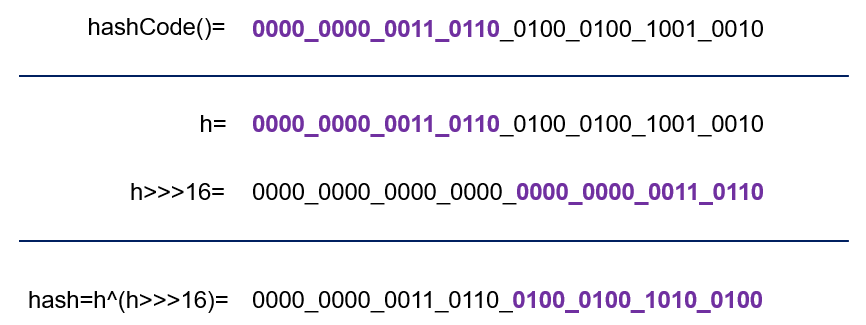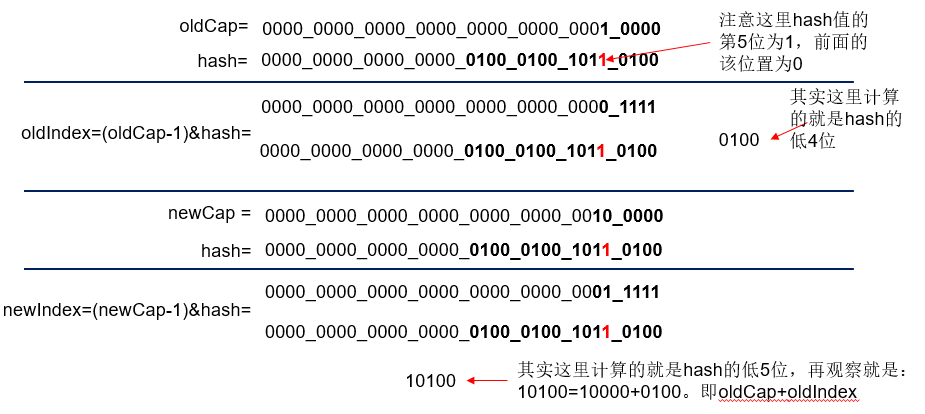# 深入理解Java中的HashMap

## 一、HashMap的結構圖示

​本文主要說的是jdk1.8版本中的實現。而1.8中HashMap是數組+鏈表+紅黑樹實現的，大概如下圖所示。後面還是主要介紹Hash Map中主要的一些成員以及方法原理。​那麼上述圖示中的結點Node具體類型是什麼，源碼如下。Node是HashMap的內部類，實現瞭Map.Entery接口，主要就是存放我們put方法所添加的元素。其中的next就表示這可以構成一個單向鏈表，這主要是通過鏈地址法解決發生hash沖突問題。而當桶中的元素個數超過閾值的時候就換轉為紅黑樹。

```//hash桶中的結點Node,實現瞭Map.Entry
static class Node<K,V> implements Map.Entry<K,V> {
final int hash;
final K key;
V value;
Node<K,V> next; //鏈表的next指針
Node(int hash, K key, V value, Node<K,V> next) {
this.hash = hash;
this.key = key;
this.value = value;
this.next = next;
}
public final K getKey()        { return key; }
public final V getValue()      { return value; }
public final String toString() { return key + "=" + value; }
//重寫Object的hashCode
public final int hashCode() {
return Objects.hashCode(key) ^ Objects.hashCode(value);
}
public final V setValue(V newValue) {
V oldValue = value;
value = newValue;
return oldValue;
}
//equals方法
public final boolean equals(Object o) {
if (o == this)
return true;
if (o instanceof Map.Entry) {
Map.Entry<?,?> e = (Map.Entry<?,?>)o;
if (Objects.equals(key, e.getKey()) &&
Objects.equals(value, e.getValue()))
return true;
}
return false;
}
}
//轉變為紅黑樹後的結點類
static final class TreeNode<k,v> extends LinkedHashMap.Entry<k,v> {
TreeNode<k,v> parent;  // 父節點
TreeNode<k,v> left; //左子樹
TreeNode<k,v> right;//右子樹
TreeNode<k,v> prev;    // needed to unlink next upon deletion
boolean red;    //顏色屬性
TreeNode(int hash, K key, V val, Node<k,v> next) {
super(hash, key, val, next);
}
//返回當前節點的根節點
final TreeNode<k,v> root() {
for (TreeNode<k,v> r = this, p;;) {
if ((p = r.parent) == null)
return r;
r = p;
}
}
}```

​上面隻是大概瞭解瞭一下HashMap的簡單組成，下面主要介紹其中的一些參數和重要的方法原理實現。

## 二、HashMap的成員變量以及含義

```//默認初始化容量初始化=16
static final int DEFAULT_INITIAL_CAPACITY = 1 << 4; // aka 16
//最大容量 = 1 << 30
static final int MAXIMUM_CAPACITY = 1 << 30;
//默認加載因子.一般HashMap的擴容的臨界點是當前HashMap的大小 > DEFAULT_LOAD_FACTOR *
//DEFAULT_INITIAL_CAPACITY = 0.75F * 16
static final float DEFAULT_LOAD_FACTOR = 0.75f;
//當hash桶中的某個bucket上的結點數大於該值的時候，會由鏈表轉換為紅黑樹
static final int TREEIFY_THRESHOLD = 8;
//當hash桶中的某個bucket上的結點數小於該值的時候，紅黑樹轉變為鏈表
static final int UNTREEIFY_THRESHOLD = 6;
//桶中結構轉化為紅黑樹對應的table的最小大小
static final int MIN_TREEIFY_CAPACITY = 64;
//hash算法,計算傳入的key的hash值，下面會有例子說明這個計算的過程
static final int hash(Object key) {
int h;
return (key == null) ? 0 : (h = key.hashCode()) ^ (h >>> 16);
}
//tableSizeFor(initialCapacity)返回大於initialCapacity的最小的二次冪數值。下面會有例子說明
static final int tableSizeFor(int cap) {
int n = cap - 1;
n |= n >>> 1;
n |= n >>> 2;
n |= n >>> 4;
n |= n >>> 8;
n |= n >>> 16;
return (n < 0) ? 1 : (n >= MAXIMUM_CAPACITY) ? MAXIMUM_CAPACITY : n + 1;
}
//hash桶
transient Node<K,V>[] table;
//保存緩存的entrySet
transient Set<Map.Entry<K,V>> entrySet;
//桶的實際元素個數 != table.length
transient int size;
//擴容或者更改瞭map的計數器。含義：表示這個HashMap結構被修改的次數，結構修改是那些改變HashMap中的映射數量或者
//修改其內部結構（例如，重新散列rehash）的修改。 該字段用於在HashMap失敗快速（fast-fail）的Collection-views
//上創建迭代器。
transient int modCount;
//臨界值，當實際大小（cap*loadFactor）大於該值的時候，會進行擴充
int threshold;
//加載因子
final float loadFactor;```

### 2.1、hash方法說明

```//hash算法
static final int hash(Object key) {
int h;
//key == null : 返回hash=0
//key != null
//（1）得到key的hashCode：h=key.hashCode()
//（2）將h無符號右移16位
//（3）異或運算：h ^ h>>>16
return (key == null) ? 0 : (h = key.hashCode()) ^ (h >>> 16);
}   ```

​假設現在我們向一個map中添加元素，例如map.put(“fsmly”,”test”)，那麼其中key為”fsmly”的hashCode的二進制表示為0000_0000_0011_0110_0100_0100_1001_0010，按照上面的步驟來計算，那麼我們調用hash算法得到的hash值為：‭### 2.2、tableSizeFor方法說明

​該方法的作用就是：返回大於initialCapacity的最小的二次冪數值。如下實例

```//n=cap-1=5; 5的二進制0101B。>>> 操作符表示無符號右移，高位取0
//n |= n>>>1: (1)n=0101 | 0101>>>1; (2)n=0101 | 0010; (3)n = 0111B
//n |= n>>>2: (1)n=0111 | 0111>>>2; (2)n=0111 | 0011; (3)n = 0111B
//n |= n>>>4: (1)n=0111 | 0111>>>4; (2)n=0111 | 0000; (3)n = 0111B
//n |= n>>>8: (1)n=0111 | 0111>>>8; (2)n=0111 | 0000; (3)n = 0111B
//n |= n>>>16:(1)n=0111 | 0111>>>16;(2)n=0111 | 0000; (3)n = 0111B
static final int tableSizeFor(int cap) {
int n = cap - 1;
n |= n >>> 1;
n |= n >>> 2;
n |= n >>> 4;
n |= n >>> 8;
n |= n >>> 16;
//n<0返回1
//n>最大容量，返回最大容量
//否則返回n+1(0111B+1B=1000B=8)
return (n < 0) ? 1 : (n >= MAXIMUM_CAPACITY) ? MAXIMUM_CAPACITY : n + 1;
}```

​再看下面這個：

```//至於這裡為什麼減1，當傳入的cap為2的整數次冪的時候，減1即保證最後的計算結果還是cap，而不是大於cap的另一個2的
//整數次冪，例如我們傳入cap=16=10000B.按照上面那樣計算
//n=cap-1=15=1111B.按照上面的方法計算得到：
// n |= n>>>1: n=1111|0111=1111;後面還是相同的結果最後n=1111B=15.
//所以返回的時候為return 15+1;
int n = cap - 1; ```

## 三、HashMap的構造方法

​我們看看HashMap源碼中為我們提供的四個構造方法。我們可以看到，平常我們最常用的無參構造器內部隻是僅僅初始化瞭loadFactor，別的都沒有做，底層的數據結構則是延遲到插入鍵值對時再進行初始化，或者說在resize中會做。後面說到擴容方法的實現的時候會講到。

```//（1）參數為初始化容量和加載因子的構造函數
public HashMap(int initialCapacity, float loadFactor) {
if (initialCapacity < 0)
throw new IllegalArgumentException("Illegal initial capacity: " +
initialCapacity);
if (initialCapacity > MAXIMUM_CAPACITY)
initialCapacity = MAXIMUM_CAPACITY;
if (loadFactor <= 0 || Float.isNaN(loadFactor))
throw new IllegalArgumentException("Illegal load factor: " +
loadFactor);
this.loadFactor = loadFactor;
this.threshold = tableSizeFor(initialCapacity); //閾值為大於initialCapacity的最小二次冪
}
//（2）隻給定初始化容量，那麼加載因子就是默認的加載因子：0.75
public HashMap(int initialCapacity) {
this(initialCapacity, DEFAULT_LOAD_FACTOR);
}
//（3）加載因子為默認的加載因子，但是這個時候的初始化容量是沒有指定的，後面調用put或者get方法的時候才resize
public HashMap() {
this.loadFactor = DEFAULT_LOAD_FACTOR; // all other fields defaulted
}
//（4）將傳遞的map中的值調用putMapEntries加入新的map集合中，其中加載因子是默認的加載因子
public HashMap(Map<? extends K, ? extends V> m) {
this.loadFactor = DEFAULT_LOAD_FACTOR;
putMapEntries(m, false);
}```

## 四、HashMap元素在數組中的位置

​不管增加、刪除、查找鍵值對，定位到哈希桶數組的索引都是很關鍵的第一步，所以我們看看源碼怎樣通過hash()方法以及其他代碼確定一個元素在hash桶中的位置的。

```//計算map中key的hash值
static final int hash(Object key) {
int h;
return (key == null) ? 0 : (h = key.hashCode()) ^ (h >>> 16);
}
//這一小段代碼就是定位元素在桶中的位置。具體做法就是：容量n-1 & hash.
//其中n是一個2的整數冪，而(n - 1) & hash其實質就是hash%n,但
//是取餘運算的效率不如位運算與，並且(n - 1) & hash也能保證散列均勻，不會產生隻有偶數位有值的現象
p = tab[i = (n - 1) & hash];```

​下面我們通過一個例子計算一下上面這個定位的過程，假設現在桶大小n為16.​我們可以看到，這裡的hash方法並不是用原有對象的hashcode最為最終的hash值，而是做瞭一定位運算，大概因為如果(n-1)的值太小的話，(n – 1) & hash的值就完全依靠hash的低位值，比如n-1為0000 1111，那麼最終的值就完全依賴於hash值的低4位瞭，這樣的話hash的高位就玩完全失去瞭作用，h ^ (h >>> 16)，通過這種方式，讓高位數據與低位數據進行異或，也是變相的加大瞭hash的隨機性，這樣就不單純的依賴對象的hashcode方法瞭。

## 五、HashMap的put方法分析

### 5.1、put方法源碼分析

```public V put(K key, V value) {
return putVal(hash(key), key, value, false, true);
}

final V putVal(int hash, K key, V value, boolean onlyIfAbsent,
boolean evict) {
Node<K,V>[] tab;  Node<K,V> p;  int n, i;
//table == null 或者table的長度為0，調用resize方法進行擴容
//這裡也說明：table 被延遲到插入新數據時再進行初始化
if ((tab = table) == null || (n = tab.length) == 0)
n = (tab = resize()).length;
// 這裡就是調用瞭Hash算法的地方，具體的計算可參考後面寫到的例子
//這裡定位坐標的做法在上面也已經說到過
if ((p = tab[i = (n - 1) & hash]) == null)
// 如果計算得到的桶下標值中的Node為null，就新建一個Node加入該位置(這個新的結點是在
//table數組中)。而該位置的hash值就是調用hash()方法計算得到的key的hash值
tab[i] = newNode(hash, key, value, null);
//這裡表示put的元素用自己key的hash值計算得到的下表和桶中的第一個位置元素產生瞭沖突，具體就是
//(1)key相同，value不同
//(2)隻是通過hash值計算得到的下標相同，但是key和value都不同。這裡處理的方法就是鏈表和紅黑樹
else {
Node<K,V> e; K k;
//上面已經計算得到瞭該hash對應的下標i，這裡p=tab[i]。這裡比較的有：
//(1)tab[i].hash是否等於傳入的hash。這裡的tab[i]就是桶中的第一個元素
//(2)比較傳入的key和該位置的key是否相同
//(3)如果都相同，說明是同一個key，那麼直接替換對應的value值(在後面會進行替換)
if (p.hash == hash && ((k = p.key) == key || (key != null && key.equals(k))))
//將桶中的第一個元素賦給e，用來記錄第一個位置的值
e = p;
//這裡判斷為紅黑樹。hash值不相等，key不相等；為紅黑樹結點
else if (p instanceof TreeNode)
e = ((TreeNode<K,V>)p).putTreeVal(this, tab, hash, key, value); //加入紅黑樹
//判斷為鏈表結點
else {
for (int binCount = 0; ; ++binCount) {
//如果達到鏈表的尾部
if ((e = p.next) == null) {
//在尾部插入新的結點
p.next = newNode(hash, key, value, null);
//前面的binCount是記錄鏈表長度的，如果該值大於8，就會轉變為紅黑樹
if (binCount >= TREEIFY_THRESHOLD - 1) // -1 for 1st
treeifyBin(tab, hash);
break;
}
//如果在遍歷鏈表的時候，判斷得出要插入的結點的key和鏈表中間的某個結點的key相
//同，就跳出循環,後面也會更新舊的value值
if (e.hash == hash &&
((k = e.key) == key || (key != null && key.equals(k))))
break;
//e = p.next。遍歷鏈表所用
p = e;
}
}
//判斷插入的是否存在HashMap中，上面e被賦值，不為空，則說明存在，更新舊的鍵值對
if (e != null) { // existing mapping for key
V oldValue = e.value;
if (!onlyIfAbsent || oldValue == null)
e.value = value; //用傳入的參數value更新舊的value值
afterNodeAccess(e);
return oldValue; //返回舊的value值
}
}
//modCount修改
++modCount;
//容量超出就擴容
if (++size > threshold)
resize();
afterNodeInsertion(evict);
return null;
}```

### 5.2、put方法執行過程總結

​可以看到主要邏輯在put方法中調用瞭putVal方法，傳遞的參數是調用瞭hash()方法計算key的hash值，主要邏輯在putVal中。可以結合註釋熟悉這個方法的執行，我在這裡大概總結一下這個方法的執行：

1.首先 (tab = table) == null || (n = tab.length) == 0這一塊判斷hash桶是否為null，如果為null那麼會調用resize方法擴容。後面我們會說到這個方法

2.定位元素在桶中的位置，具體就是通過key的hash值和hash桶的長度計算得到下標i，如果計算到的位置處沒有元素(null)，那麼就新建結點然後添加到該位置。

3.如果table[i]處不為null，已經有元素瞭，那麼就表明產生hash沖突,這裡可能是三種情況

①判斷key是不是一樣，如果key一樣，那麼就將新的值替換舊的值；

②如果不是因為key一樣，那麼需要判斷當前該桶是不是已經轉為瞭紅黑樹，是的話就構造一個TreeNode結點插入紅黑樹；

③不是紅黑樹，就使用鏈地址法處理沖突問題。這裡主要就是遍歷鏈表，如果在遍歷過程中也找到瞭key一樣的元素，那麼久還是使用新值替換舊值。否則會遍歷到鏈表結尾處，到這裡就直接新添加一個Node結點插入鏈表，插入之後還需要判斷是不是已將超過瞭轉換為紅黑樹的閾值8，如果超過就會轉為紅黑樹。

4.最後需要修改modCount的值。

5.判斷插入後的size大小是不是超過瞭threshhold，如果超過需要進行擴容。

## 六、HashMap的resize方法分析

### 6.1、resize方法源碼

​擴容(resize)就是重新計算容量，具體就是當map內部的size大於DEFAULT_LOAD_FACTOR * DEFAULT_INITIAL_CAPACITY ，就需要擴大數組的長度，以便能裝入更多的元素。resize方法實現中是使用一個新的數組代替已有的容量小的數組。

```//該方法有2種使用情況：1.初始化哈希表(table==null) 2.當前數組容量過小，需擴容
final Node<K,V>[] resize() {
Node<K,V>[] oldTab = table; //oldTab指向舊的table數組
//oldTab不為null的話，oldCap為原table的長度
//oldTab為null的話，oldCap為0
int oldCap = (oldTab == null) ? 0 : oldTab.length;
int oldThr = threshold; //閾值
int newCap, newThr = 0;
if (oldCap > 0) {
//這裡表明oldCap！=0，oldCap=原table.length();
if (oldCap >= MAXIMUM_CAPACITY) {
threshold = Integer.MAX_VALUE; //如果大於最大容量瞭，就賦值為整數最大的閥值
return oldTab;
}
// 如果數組的長度在擴容後小於最大容量 並且oldCap大於默認值16(這裡的newCap也是在原來的
//長度上擴展兩倍)
else if ((newCap = oldCap << 1) < MAXIMUM_CAPACITY &&
oldCap >= DEFAULT_INITIAL_CAPACITY)
newThr = oldThr << 1; // double threshold雙倍擴展threshhold
}
else if (oldThr > 0) // initial capacity was placed in threshold
//	這裡的oldThr=tabSizeFor(initialCapacity),從上面的構造方法看出，如果不是調用的
//無參構造，那麼threshhold肯定都會是經過tabSizeFor運算得到的2的整數次冪的,所以可以將
//其作為Node數組的長度(個人理解)
newCap = oldThr;
else { // zero initial threshold signifies using defaults(零初始閾值表示使用默認值)
//這裡說的是我們調用無參構造函數的時候(table == null,threshhold = 0)，新的容量等於默
//認的容量，並且threshhold也等於默認加載因子*默認初始化容量
newCap = DEFAULT_INITIAL_CAPACITY;
newThr = (int)(DEFAULT_LOAD_FACTOR * DEFAULT_INITIAL_CAPACITY);
}
// 計算新的resize上限
if (newThr == 0) {
float ft = (float)newCap * loadFactor; //容量 * 加載因子
newThr = (newCap < MAXIMUM_CAPACITY && ft < (float)MAXIMUM_CAPACITY ?
(int)ft : Integer.MAX_VALUE);
}
threshold = newThr;
@SuppressWarnings({"rawtypes","unchecked"})
//以新的容量作為長度，創建一個新的Node數組存放結點元素
//當然，桶數組的初始化也是在這裡完成的
Node<K,V>[] newTab = (Node<K,V>[])new Node[newCap];
table = newTab;
//原來的table不為null
if (oldTab != null) {
// 把每個bucket都移動到新的buckets中
for (int j = 0; j < oldCap; ++j) {
Node<K,V> e;
//原table中下標j位置不為null
if ((e = oldTab[j]) != null) {
oldTab[j] = null; //將原來的table[j]賦為null，及時GC？
if (e.next == null) //如果該位置沒有鏈表，即隻有數組中的那個元素
//通過新的容量計算在新的table數組中的下標：(n-1)&hash
newTab[e.hash & (newCap - 1)] = e;
else if (e instanceof TreeNode)
//如果是紅黑樹結點，重新映射時，需要對紅黑樹進行拆分
((TreeNode<K,V>)e).split(this, newTab, j, oldCap);
else { // 鏈表優化重hash的代碼塊
Node<K,V> loHead = null, loTail = null;
Node<K,V> hiHead = null, hiTail = null;
Node<K,V> next;
do {//上面判斷不是紅黑樹，那就是鏈表，這裡就遍歷鏈表，進行重新映射
next = e.next;
// 原位置
if ((e.hash & oldCap) == 0) {
//loTail處為null，那麼直接加到該位置
if (loTail == null)
loHead = e;
//loTail為鏈表尾結點，添加到尾部
else
loTail.next = e;
//添加後，將loTail指向鏈表尾部，以便下次從尾部添加
loTail = e;
}
// 原位置+舊容量
else {
//hiTail處為null，就直接點添加到該位置
if (hiTail == null)
hiHead = e;
//hiTail為鏈表尾結點，尾插法添加
else
hiTail.next = e;
hiTail = e;
}
} while ((e = next) != null);
// 將分組後的鏈表映射到新桶中
// 原索引放到bucket裡
if (loTail != null) {
//舊鏈表遷移新鏈表,鏈表元素相對位置沒有變化;
//實際是對對象的內存地址進行操作
loTail.next = null;//鏈表尾元素設置為null
newTab[j] = loHead; //數組中位置為j的地方存放鏈表的head結點
}
// 原索引+oldCap放到bucket裡
if (hiTail != null) {
hiTail.next = null;
newTab[j + oldCap] = hiHead;
}
}
}
}
}
return newTab;
}```

### 6.2、(e.hash & oldCap) == 0分析

​我這裡添加上一點，就是為什麼使用 (e.hash & oldCap) == 0判斷是處於原位置還是放在更新的位置（原位置+舊容量），解釋如下：我們知道capacity是2的冪，所以oldCap為10…0的二進制形式(比如16=10000B)。

（1）若判斷條件為真，意味著oldCap為1的那位對應的hash位為0(1&0=0,其他位都是0,結果自然是0)，對新索引的計算沒有影響，至於為啥沒影響下面就說到瞭。先舉個例子計算一下數組中的下標在擴容前後的變化：​從上面計算發現，當cap為1的那位對應的hash為0的時候，resize前後的index是不變的。我們再看下面，使用上面的hash值，對應的就是 (e.hash & oldCap) == 0，恰好也是下標不變的​（2）若判斷條件為假，則 oldCap為1的那位對應的hash位為1。比如新下標=hash&( newCap-1 )= hash&( (16<<2) – 1)=10010，相當於多瞭10000，即 oldCap .如同下面的例子​從上面計算發現，當cap為1的那位對應的hash為1的時候，resize前後的index是改變的。我們再看下面，使用上面的hash值，對應的就是 (e.hash & oldCap) != 0，恰好下標就是原索引+原容量### 6.3、部分代碼理解

​這一部分其實和put方法中，使用鏈地址法解決hash沖突的原理差不多，都是對鏈表的操作。

```// 原位置
if ((e.hash & oldCap) == 0) {
//loTail處為null，那麼直接加到該位置
if (loTail == null)
loHead = e;
//loTail為鏈表尾結點，添加到尾部
else
loTail.next = e;
//添加後，將loTail指向鏈表尾部，以便下次從尾部添加
loTail = e;
}
// 原位置+舊容量
else {
//hiTail處為null，就直接點添加到該位置
if (hiTail == null)
hiHead = e;
//hiTail為鏈表尾結點，尾插法添加
else
hiTail.next = e;
hiTail = e;
}```

​我們直接通過一個簡單的圖來理解吧### 6.4、resize總結

​resize代碼稍微長瞭點，但是總結下來就是這幾點

## 七、HashMap的get方法分析

### 7.1、get方法源碼

​基本邏輯就是根據key算出hash值定位到哈希桶的索引，當可以就是當前索引的值則直接返回其對於的value，反之用key去遍歷equal該索引下的key，直到找到位置。

```public V get(Object key) {
Node<K,V> e;
return (e = getNode(hash(key), key)) == null ? null : e.value;
}

final Node<K,V> getNode(int hash, Object key) {
Node<K,V>[] tab; Node<K,V> first, e; int n; K k;
//計算存放在數組table中的位置.具體計算方法上面也已經介紹瞭
if ((tab = table) != null && (n = tab.length) > 0 &&
(first = tab[(n - 1) & hash]) != null) {
//先查找是不是就是數組中的元素
if (first.hash == hash && // always check first node
((k = first.key) == key || (key != null && key.equals(k))))
return first;
//該位置為紅黑樹根節點或者鏈表頭結點
if ((e = first.next) != null) {
//如果first為紅黑樹結點，就在紅黑樹中遍歷查找
if (first instanceof TreeNode)
return ((TreeNode<K,V>)first).getTreeNode(hash, key);
//不是樹結點，就在鏈表中遍歷查找
do {
if (e.hash == hash &&
((k = e.key) == key || (key != null && key.equals(k))))
return e;
} while ((e = e.next) != null);
}
}
return null;
}```